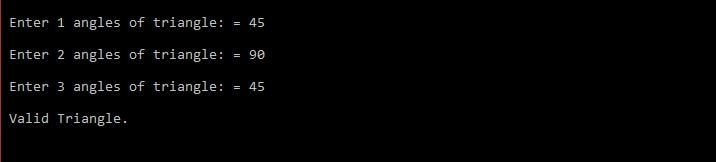# C program to check whether the triangle is valid or not if angles are given

Before writing the C program to check whether a triangle is valid or not if angles are given, we should know the properties of triangles. The angle property of the triangle says that the sum of all three angles should be equal to 180.

#include <stdio.h>
int main()
{
//variable to store angles
int angle1, angle2, angle3, sum;
// Get three angles of triangle from the user
printf(“\n Enter 1 angles of triangle: = “);
scanf(“%d”, &angle1);
printf(“\n Enter 2 angles of triangle: = “);
scanf(“%d”, &angle2);
printf(“\n Enter 3 angles of triangle: = “);
scanf(“%d”, &angle3);
//Calculate sum of angles
sum = angle1 + angle2 + angle3;
//check sum of three angles
if(sum == 180 && angle1 != 0 && angle2 != 0 && angle3 != 0)
{
printf(“\n Valid Triangle.\n\n”);
}
else
{
printf(“\n Not valid Triangle.\n\n”);
}
return 0;
}

Output:

TOP